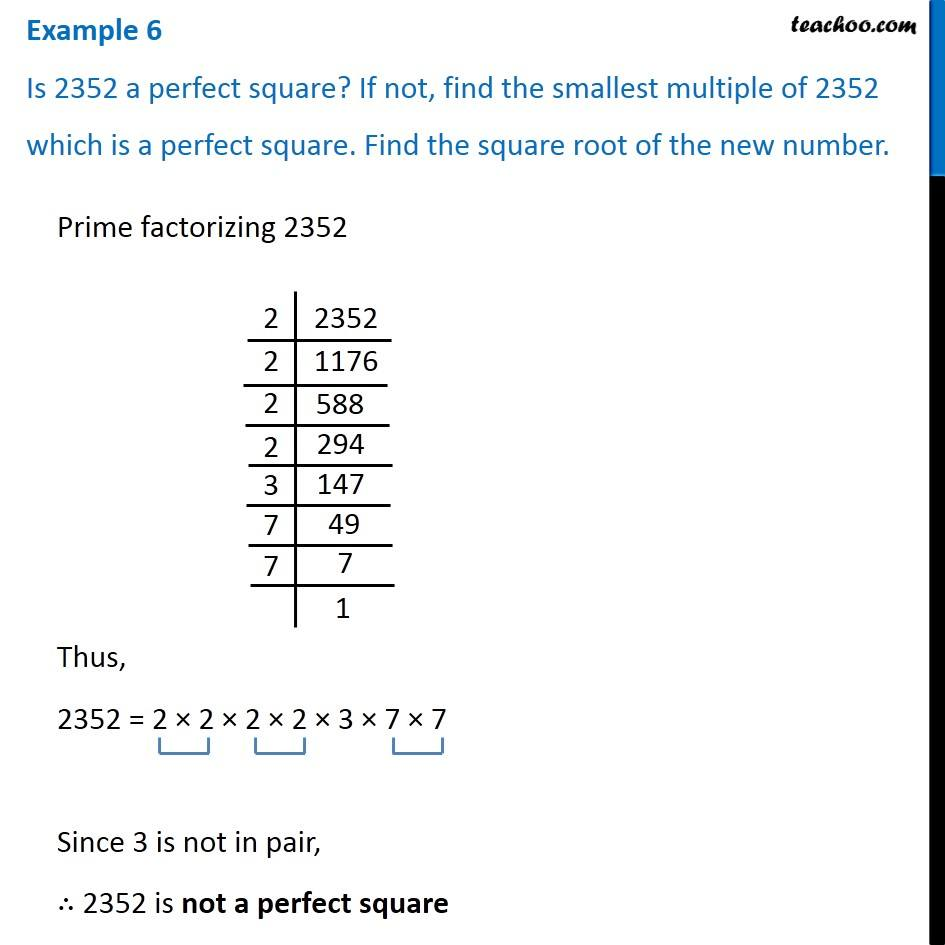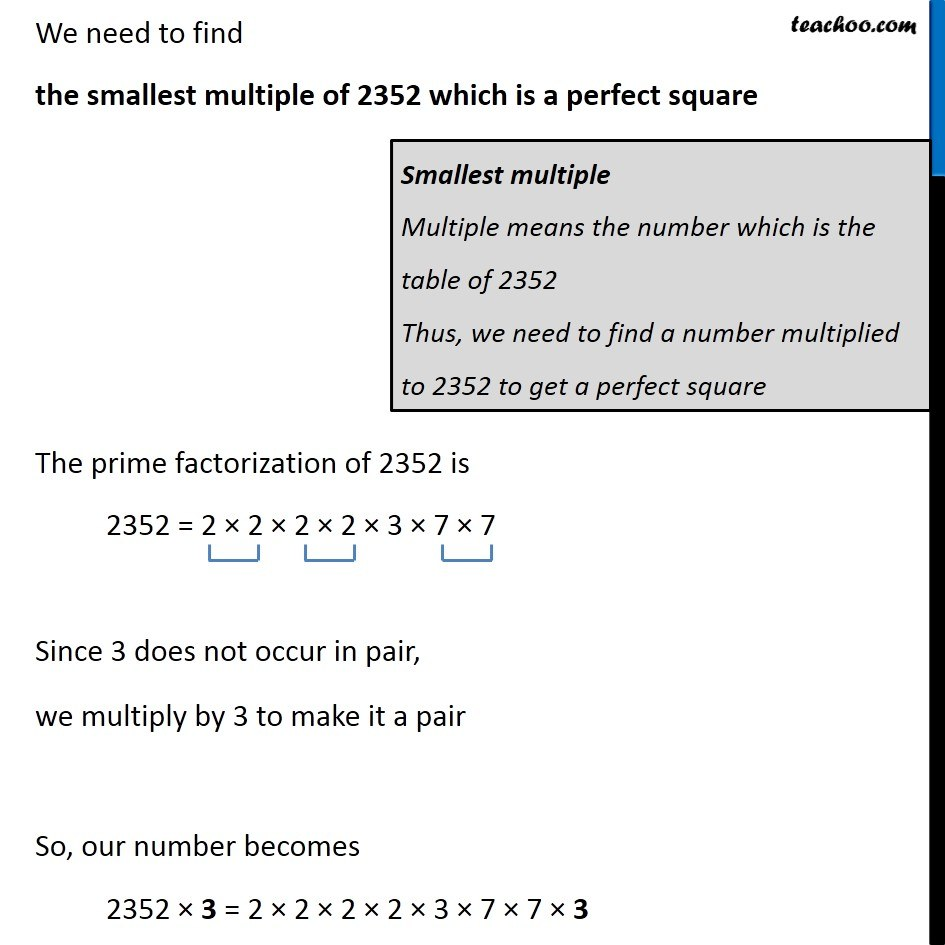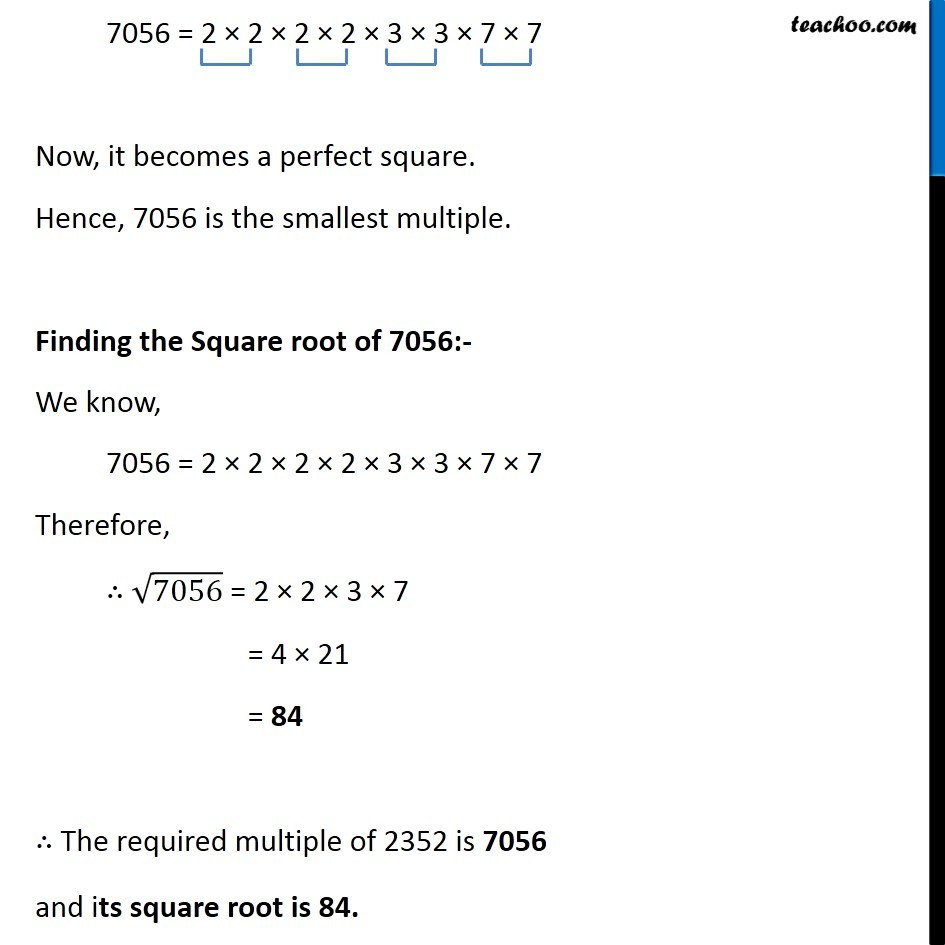Subscribe to our Youtube Channel - https://you.tube/teachoo

1. Chapter 6 Class 8 Squares and Square Roots
2. Concept wise
3. Smallest number multiplied to get perfect square

Transcript

Example 6 Is 2352 a perfect square? If not, find the smallest multiple of 2352 which is a perfect square. Find the square root of the new number.Prime factorizing 2352 Thus, 2352 = 2 × 2 × 2 × 2 × 3 × 7 × 7 Since 3 is not in pair, ∴ 2352 is not a perfect square We need to find the smallest multiple of 2352 which is a perfect square The prime factorization of 2352 is 2352 = 2 × 2 × 2 × 2 × 3 × 7 × 7 Since 3 does not occur in pair, we multiply by 3 to make it a pair So, our number becomes 2352 × 3 = 2 × 2 × 2 × 2 × 3 × 7 × 7 × 3 Smallest multiple Multiple means the number which is the table of 2352 Thus, we need to find a number multiplied to 2352 to get a perfect square 7056 = 2 × 2 × 2 × 2 × 3 × 3 × 7 × 7 Now, it becomes a perfect square. Hence, 7056 is the smallest multiple. Finding the Square root of 7056:- We know, 7056 = 2 × 2 × 2 × 2 × 3 × 3 × 7 × 7 Therefore, ∴ √7056 = 2 × 2 × 3 × 7 = 4 × 21 = 84 ∴ The required multiple of 2352 is 7056 and its square root is 84.

Smallest number multiplied to get perfect square

About the AuthorDavneet Singh
Davneet Singh is a graduate from Indian Institute of Technology, Kanpur. He has been teaching from the past 9 years. He provides courses for Maths and Science at Teachoo.